Courses

Test: Single Correct MCQs: Units and Measurements | JEE Advanced

15 Questions MCQ Test Physics 35 Years JEE Main & Advanced Past year Papers | Test: Single Correct MCQs: Units and Measurements | JEE Advanced

Description
Attempt Test: Single Correct MCQs: Units and Measurements | JEE Advanced | 15 questions in 30 minutes | Mock test for JEE preparation | Free important questions MCQ to study Physics 35 Years JEE Main & Advanced Past year Papers for JEE Exam | Download free PDF with solutions
QUESTION: 1

The dimension of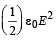(ε0 : permittivity of free space, Eelectric field)

Solution:

Note : Hererepresents energy per unit volume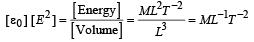QUESTION: 2

A quantity X is given by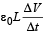where ∈0 is the permittivity of the free space, L is a length, ΔV is a potential difference and Δt is a time interval. The dimensional formula for X is the same as that of

Solution:

Dimensionally ε0L= Capacitance (c)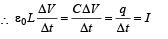QUESTION: 3

A cube has a side of length 1.2 × 10–2m. Calculate its volume.

Solution: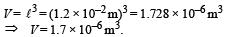QUESTION: 4

Pressure depends on distance as,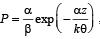, where α, β are constants, z is distance, k is Boltzman’s constant and θ is temperature. The dimension of β are

Solution:

Unit of k is joules per kelvin or dimensional formula of k is [ML2T–2 θ -1] Note : The power of an exponent is a number.

Therefore, dimensionally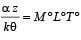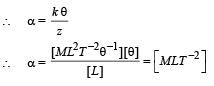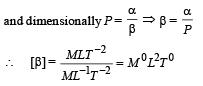QUESTION: 5

A wire of length ℓ = 6 ± 0.06 cm and radius r = 0.5 ± 0.005 cm and mass m = 0.3 ± 0.003 gm. Maximum percentage error in density is

Solution: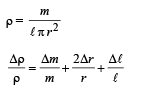Putting the values Δℓ = 0.06 cm, ℓ = 6 cm; Δr = 0.005 cm; r = 0.5 cm,
m = 0.3 gm; Δm = 0.003 gm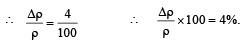QUESTION: 6

Which of the following set have different dimensions?

Solution:

Electric flux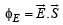∴ Dimensionally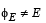QUESTION: 7

In a screw gauge, the zero of mainscale coincides with fifth division of circular scale in figure (i). The circular division of screw gauge are 50. It moves 0.5 mm on main scale in one rotation. The diameter of the ball in figure (ii) is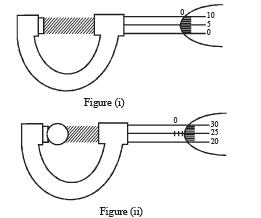Solution:

Least count = 0.5/50 = 0.01mm

Zero error = 5 × L.C = 5 × 0.01 mm = 0.05 mm
Diameter of ball = [Reading on main scale] + [Reading on circular scale × L . C] – Zero error = 0.5 × 2 + 25 × 0.01 – 0.05  = 1.20 mm

QUESTION: 8

A student performs an exper iment for determination of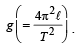The error in length ℓ is Δℓ and in time T is ΔT and n is number of times the reading is taken. The measurement of g is most accurate for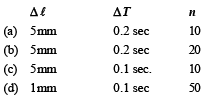Solution: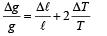Δℓ and ΔT are least and number of readings are maximum in option (d), therefore the measurement of g is most accurate with data used in this option.

QUESTION: 9

A student performs an experiment to determine the Young's modulus of a wire, exactly 2 m long, by Searle's method. In a particular reading, the student measures the extension in the length of the wire to be 0.8 mm with an uncertainty of ± 0.05 mm at a load of exactly 1.0 kg. The student also measures the diameter of the wire to be 0.4 mm with an uncertainty of ± 0.01 mm. Take g = 9.8 m/s2 (exact). The Young's modulus obtained from the reading is

Solution: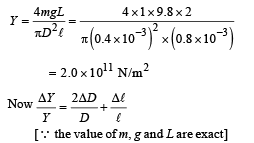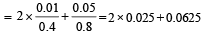= 0.05 + 0.0625 = 0.1125
⇒ ΔY = 2 × 1011 × 0.1125 = 0.225 × 1011
= 0.2 × 1011 N/m2

Note: We can also take value of y from options given without calculating it as it is same in all options.

∴ Y = ( 2 ± 0.2) × 1011 N/m2

QUESTION: 10

Students I, II and III perform an experiment for measuring the acceleration due to gravity (g) using a simple pendulum.
They use different lengths of the pendulum and /or record time for different number of oscillations. The observations are shown in the table.

Least count for length = 0.1 cm

Least count for time = 0.1 s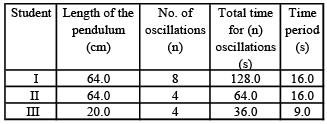If EI, EII and EIII are the percentage errors in g, i.e.,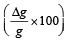for students I, II and III, respectively, then

Solution:

The time period of a simple pendulum is given by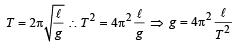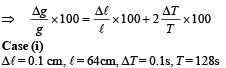QUESTION: 11

A vernier calipers has 1 mm marks on the main scale. It has 20 equal divisions on the Vernier scale which match with 16 main scale divisions. For this Vernier calipers, the least count is

Solution:

20 divisions on the vernier scale = 16 divisions of main scale

∴ 1 division on the vernier scale

= 16/20 divisions of main scale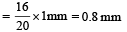We know that least count = 1MSD – 1VSD
= 1 mm – 0.8 mm  = 0.2 mm

QUESTION: 12

Th e den sity of a solid ball is to be deter mined in an experiment. The diameter of the ball is measured with a screw gauge, whose pitch is 0.5 mm and there are 50 divisions on the circular scale. The reading on the main scale is 2.5 mm and that on the circular scale is 20 divisions. If the measured mass of the ball has a relative error of 2 %, the relative percentage error in the density is

Solution:

Diameter D = M.S.R. + (C.S.R) × L.C.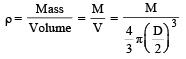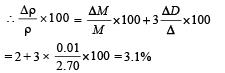QUESTION: 13

In the determination of Young’s modulus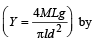using Searle’s method, a wire of length L = 2 m and diameter d = 0.5 mm is used. For a load M = 2.5 kg, an extension l = 0.25 mm in the length of the wire is observed. Quantities d and l are measured using a screw gauge and a micrometer, respectively. They have the same pitch of 0.5 mm. The number of divisions on their circular scale is 100. The contributions to the maximum probable error of the Y measurement

Solution:

The maximum possible error in Y due to l and d are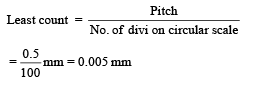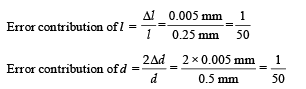QUESTION: 14

The diameter of a cylinder is measured using a Vernier callipers with no zero error. It is found that the zero of the Vernier scale lies between 5.10 cm and 5.15 cm of the main scale. The Vernier scale has 50 divisions equivalent to 2.45 cm. The 24th division of the Vernier scale exactly coincides with one of the main scale divisions. The diameter of the cylinder is

Solution:

Reading = M.S.R + No of division of V.S matching the main scale division (1MSD – 1VSD)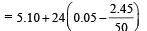= 5.124 cm    Option (b) is correct.

QUESTION: 15

There are two Vernier calipers both of which have 1 cm divided into 10 equal divisions on the main scale. The Vernier scale of one of the calipers (C1) has 10 equal divisions that correspond to 9 main scale divisions. The Vernier scale of the other caliper (C2) has 10 equal divisions that correspond to 11 main scale divisions. The readings of the two calipers are shown in the figure. The measured values (in cm) by calipers C1 and C2, respectively, are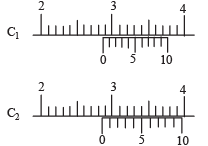Solution: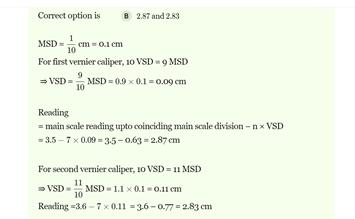Use Code STAYHOME200 and get INR 200 additional OFF Use Coupon Code

Track your progress, build streaks, highlight & save important lessons and more!

Similar ContentRelated tests